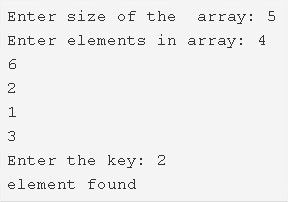# C Program To Search An Element In An Array | C Programs

C Program to search for an element in an array – In this article, we will detail in on the various methods to search for an element in an array in C programming.

Suitable examples and sample programs have also been added so that you can understand the whole thing very clearly. The compiler has also been added with which you can execute it yourself.

The means used in this specific piece are as follows:

• Using Standard Method
• Using Function
• Using Recursion

As we all know, an array is a collection or sequential arrangement of elements in any given order. Arrays form the basics of the C programming language.As you can see in the photo uploaded above, the elements are first entered in no particular order.

The elements entered here are 4, 6, 2, 1 and 3.

The target element is mentioned here as well. The target element here is 2.

Once the element is present in the array, it will say “element found”.

Thus, the several methods to find an element in an array are as follows:

## Using Standard Method

1. Read the array size and store that value into the variable n.

2) Read the entered elements and store those elements into an array a[] using scanf statement and the for loop. scanf(“%d”,&a[i]) indicates scanf statement reads the entered element and assigned that element to a[i].

3) Read the key which we want to search in an array.

4) Compare the key with each element of the array as a[i]==key and print element found, if the key matches with any element of the array otherwise print element not found.

Output:

### Using Function – Search An Element In An Array

1. The search() function compares each element of an array with the key which we want to search using if condition and the for loop.If any element matches with that key then this function returns 1, otherwise, it returns 0 to main() function at ‘if” condition.

2) The search() function will be called by passing an array, array size, key as arguments.

3) If the function search() returns 1 to if condition,then if(1) is true and prints “element found”.If it returns 0 then if(0) is false and prints “element not found”.

Output:

#### Using Recursion – Search An Element In An Array

1. In the main() search() function will be called by passing an array,0,array size,key as arguments in if condition.

2) The search() function checks the if condition i<n.If this condition is true then

a) It checks the a[i]==key,if key matches with a[i] then this function returns 1 to main() at if condition.

b) If key not matches with a[i] then it returns the same function by increasing i value as search(a,i+1,n,key) to main(). Again the search() will be called.Repeats until the key matches with any element of the array.

c) If the function returns 1 then main() prints “element found”.Otherwise, it prints “element not found”.

3) In this program search() function is recursive function.

Output: